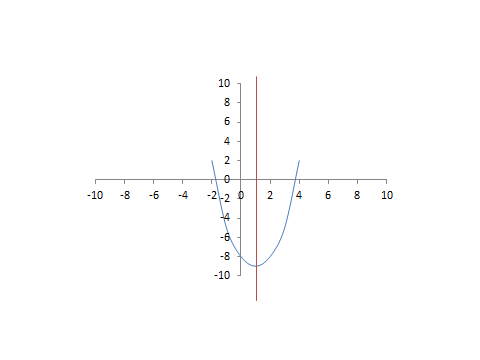# Find the Axis Of Symmetry: Equation, Formula & Vertex

Instructions:

Choose an answer and hit 'next'. You will receive your score and answers at the end.

question 1 of 3

### What is the equation of the axis of symmetry for the graph?Create Your Account To Take This Quiz

As a member, you'll also get unlimited access to over 75,000 lessons in math, English, science, history, and more. Plus, get practice tests, quizzes, and personalized coaching to help you succeed.

Try it risk-free for 30 days. Cancel anytime.

### 2. What is the axis of symmetry for a parabola expressed by the quadratic equation of 2x2 - 8x + 11?

Create your account to access this entire worksheet
A Premium account gives you access to all lesson, practice exams, quizzes & worksheets
Quizzes, practice exams & worksheets
Certificate of Completion
Create an account to get started

By supplying the answers to the multiple practice problems this quiz/worksheet will ask you, you will demonstrate your ability to identify the equations of axes of symmetry on graphs and in parabolas. Additionally, you will be asked about ordered pairs.

## Quiz & Worksheet Goals

To be clear, you will answer questions on:

• A graph's equation for the axis of symmetry
• The axis of symmetry for parabolas based on quadratic equations
• Ordered pairs of a parabola's vertex based on quadratic equations
• Finding a missing ordered pair based on a parabola's axis of symmetry

## Skills Practiced

• Knowledge application - use your knowledge to answer questions about finding axes of symmetry
• Information recall - access the knowledge you've gained regarding mathematical necessities for the axis of symmetry
• Interpreting information - verify that you can read information regarding the application of quadratic equations and interpret it correctly

You've got a chance to learn more on finding the axis of symmetry with the assistance of the tutorial, Find the Axis Of Symmetry: Equation, Formula & Vertex. This lesson will walk you through concepts like:

• The concept of symmetry
• A parabola's axis of symmetry
• The quadratic form of a parabola
• Applications of the quadratic equation
• Uses of the axis of symmetry equation
Final ExamCollege Algebra: Help and Review
Status: Not Started
Chapter ExamUsing FOIL, Graphing Parabolas & Solving Quadratics to Factor: Help & Review
Status: Not Started

Support